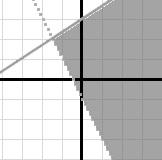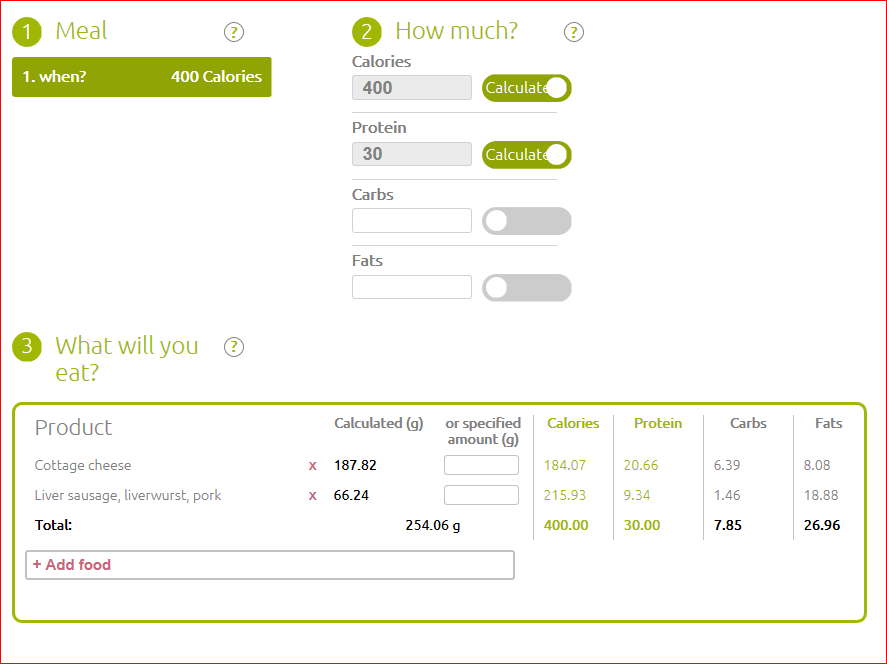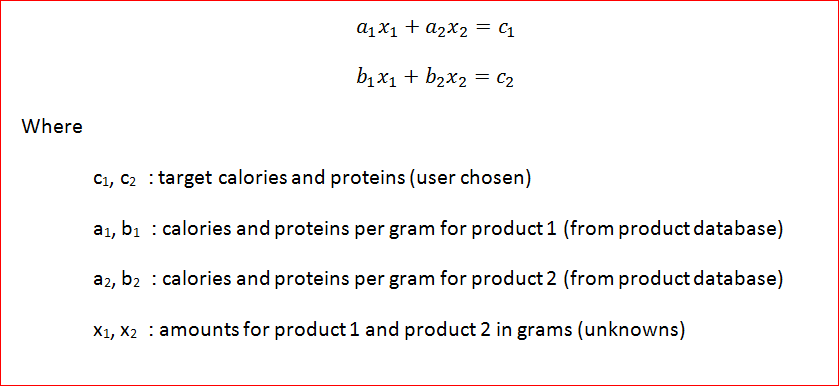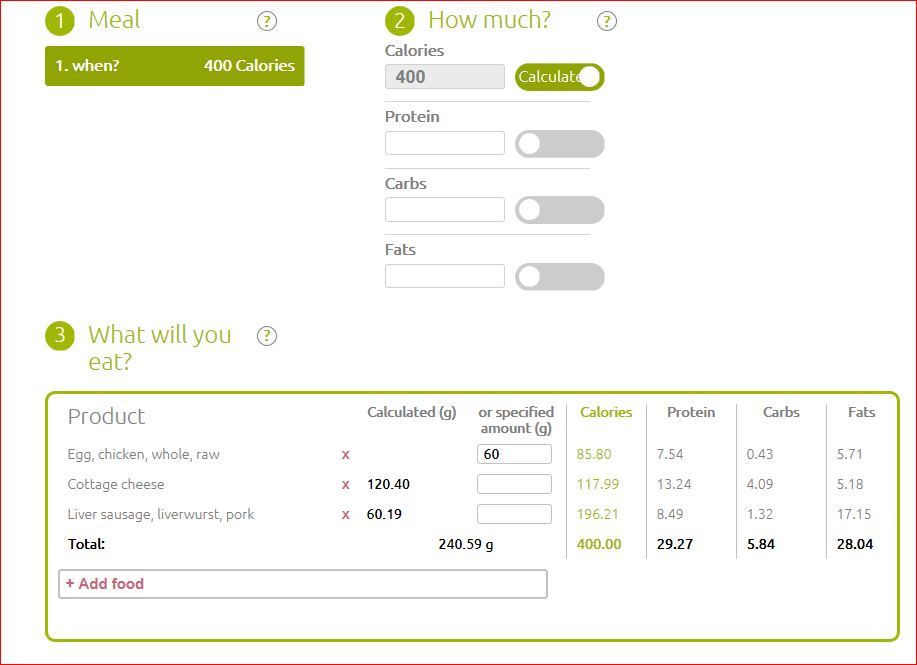Linear programming
2017/03/03   Raivis Spēlmanis

How to find generic solution of linear equation system with arbitrary number of equation and unknowns programmatically.

### Healthy diet portal

When I studied at University I thought math would never be a really useful skill. I saw mathematical analysis and linear algebra courses more of a mental exercise to help development of logical reasoning.

15 years later, when our client ordered development of calculation logic for his portal eatfridge.com, it seemed quite simple at the first glance. Users should be able to choose list of products for a meal, set the goals (calories, proteins and fats in any combination) and portal must calculate amount of each product in grams to meet set goals.### Let's remember some math

When I analyzed the problem, I tried to convert the problem into a system of linear equations:If we have 2 products and 2 goals, then this system has a definite solution. Similarly, in case of 3 products and 3 goals. And so on.

### It's not that simple

But what if user sets 1 goal and chooses 3 products? Then we have 1 equation with 2 unknowns. It gives an infinite number of solutions.And what if user sets 2 goals and chooses 1 product? There may be no solution at all.

Moreover, user should be able specify exact amount for each product. So, several unknowns may turn into constants.

How to find a generic solution for this problem?